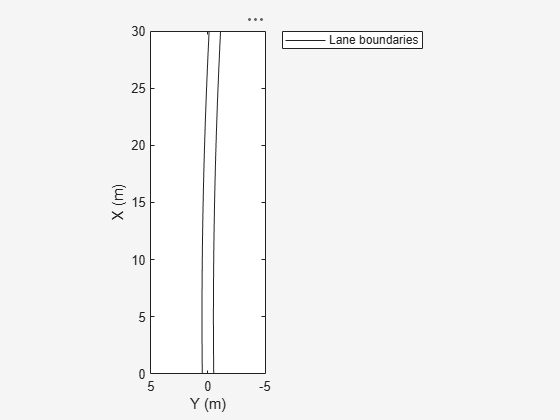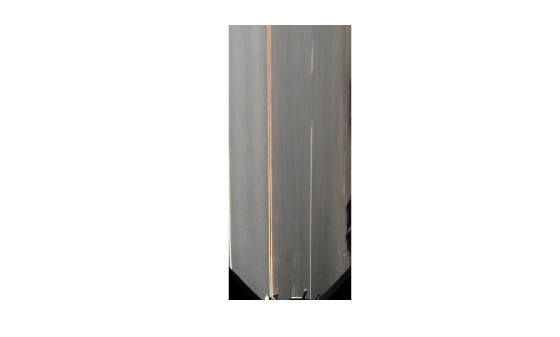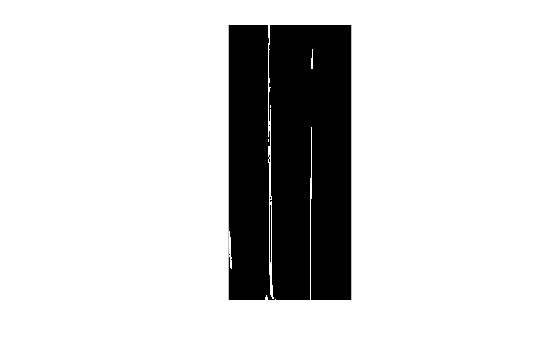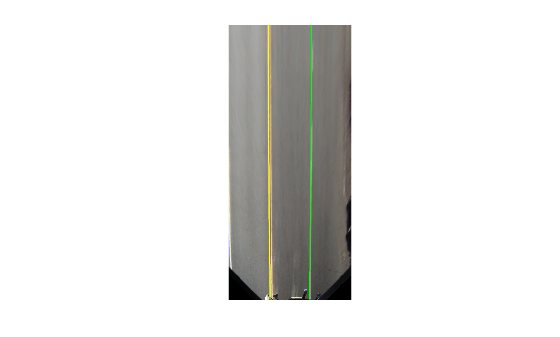Documentation

# parabolicLaneBoundary

Parabolic lane boundary model

## Description

The `parabolicLaneBoundary` object contains information about a parabolic lane boundary model.

## Creation

To generate parabolic lane boundary models that fit a set of boundary points and an approximate width, use the `findParabolicLaneBoundaries` function. If you already know your parabolic parameters, create lane boundary models by using the `parabolicLaneBoundary` function (described here).

### Syntax

``boundaries = parabolicLaneBoundary(parabolicParameters)``

### Description

example

````boundaries = parabolicLaneBoundary(parabolicParameters)` creates an array of parabolic lane boundary models from an array of ```[A B C]``` parameters for the parabolic equation y = Ax2 + Bx + C. Points within the lane boundary models are in world coordinates.```

### Input Arguments

expand all

Coefficients for parabolic models of the form y = Ax2 + Bx + C, specified as an `[A B C]` real-valued vector or as a matrix of `[A B C]` values. Each row of `parabolicParameters` describes a separate parabolic lane boundary model.

## Properties

expand all

Coefficients for a parabolic model of the form y = Ax2 + Bx + C, specified as an `[A B C]` real-valued vector.

Type of boundary, specified as a `LaneBoundaryType` of supported lane boundaries. The supported lane boundary types are:

• `Unmarked`

• `Solid`

• `Dashed`

• `BottsDots`

• `DoubleSolid`

Specify a lane boundary type as `LaneBoundaryType.BoundaryType`. For example:

```LaneBoundaryType.BottsDots ```

Strength of the boundary model, specified as a real scalar. `Strength` is the ratio of the number of unique x-axis locations on the boundary to the length of the boundary specified by the `XExtent` property. A solid line without any breaks has a higher strength than a dotted line that has breaks along the full length of the boundary.

Length of the boundary along the x-axis, specified as a ```[minX maxX]``` real-valued vector that describes the minimum and maximum x-axis locations.

## Object Functions

 `computeBoundaryModel` Obtain y-coordinates of lane boundaries given x-coordinates

## Examples

collapse all

Create left-lane and right-lane parabolic boundary models.

```llane = parabolicLaneBoundary([-0.001 0.01 0.5]); rlane = parabolicLaneBoundary([-0.001 0.01 -0.5]);```

Create a bird's-eye plot and lane boundary plotter. Plot the lane boundaries.

```bep = birdsEyePlot('XLimits',[0 30],'YLimits',[-5 5]); lbPlotter = laneBoundaryPlotter(bep,'DisplayName','Lane boundaries'); plotLaneBoundary(lbPlotter, [llane rlane]);```Find lanes in an image by using parabolic lane boundary models. Overlay the identified lanes on the original image and on a bird's-eye-view transformation of the image.

Load an image of a road with lanes. The image was obtained from a camera sensor mounted on the front of a vehicle.

`I = imread('road.png');`

Transform the image into a bird's-eye-view image by using a preconfigured sensor object. This object models the sensor that captured the original image.

```bevSensor = load('birdsEyeConfig'); birdsEyeImage = transformImage(bevSensor.birdsEyeConfig,I); imshow(birdsEyeImage)```Set the approximate lane marker width in world units (meters).

`approxBoundaryWidth = 0.25;`

Detect lane features and display them as a black-and-white image.

```birdsEyeBW = segmentLaneMarkerRidge(rgb2gray(birdsEyeImage), ... bevSensor.birdsEyeConfig,approxBoundaryWidth); imshow(birdsEyeBW)```Obtain lane candidate points in world coordinates.

```[imageX,imageY] = find(birdsEyeBW); xyBoundaryPoints = imageToVehicle(bevSensor.birdsEyeConfig,[imageY,imageX]);```

Find lane boundaries in the image by using the `findParabolicLaneBoundaries` function. By default, the function returns a maximum of two lane boundaries. The boundaries are stored in an array of `parabolicLaneBoundary` objects.

`boundaries = findParabolicLaneBoundaries(xyBoundaryPoints,approxBoundaryWidth);`

Use `insertLaneBoundary` to overlay the lanes on the original image. The `XPoints` vector represents the lane points, in meters, that are within range of the ego vehicle's sensor. Specify the lanes in different colors. By default, lanes are yellow.

```XPoints = 3:30; figure sensor = bevSensor.birdsEyeConfig.Sensor; lanesI = insertLaneBoundary(I,boundaries(1),sensor,XPoints); lanesI = insertLaneBoundary(lanesI,boundaries(2),sensor,XPoints,'Color','green'); imshow(lanesI)```View the lanes in the bird's-eye-view image.

```figure BEconfig = bevSensor.birdsEyeConfig; lanesBEI = insertLaneBoundary(birdsEyeImage,boundaries(1),BEconfig,XPoints); lanesBEI = insertLaneBoundary(lanesBEI,boundaries(2),BEconfig,XPoints,'Color','green'); imshow(lanesBEI)```